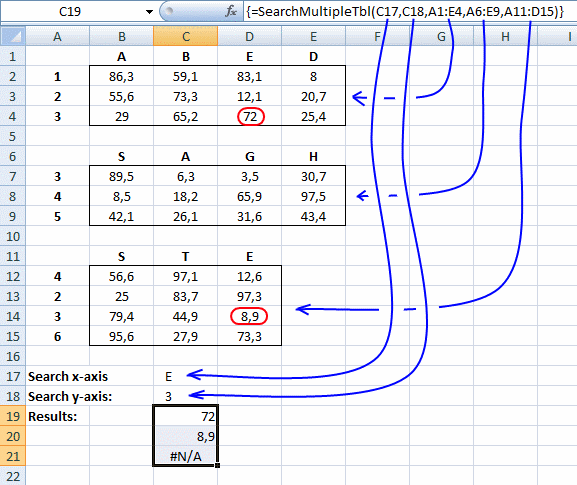Author: Oscar Cronquist Article last updated on March 28, 2023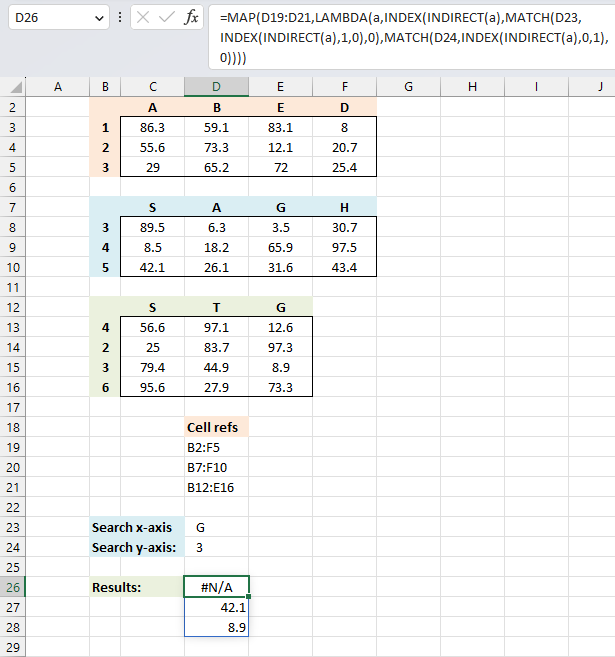This article describes two ways to perform lookups in multiple in multiple cross reference tables simultaneously. The first one being an Excel 365 LAMBDA function and the second way a User Defined Function based on Visual Basic for Applications (VBA).

## 1. Two-way lookup using multiple tables - Excel 365 LAMBDA functionThis example demonstrates how to perform lookups in multiple in multiple cross reference tables simultaneously using an Excel 365 LAMBDA function.

The first and second conditions are in cells D23 and D24 respectively. The cell references populates cells D19 to D21. The result is shown in cell D26, it spills values to cells below as far as needed.

Excel 365 formula in cell D26:

=MAP(D19:D21,LAMBDA(a,INDEX(INDIRECT(a),MATCH(D23,INDEX(INDIRECT(a),1,0),0),MATCH(D24,INDEX(INDIRECT(a),0,1),0))))

The formula returns a value for each cell reference, an #N/A error is displayed if at least one condition is not found.

### Explaining formula

#### Step 1 - Convert string to cell reference

The INDIRECT function returns the cell reference based on a text string and shows the content of that cell reference.

Function syntax: INDIRECT(ref_text, [a1])

INDIRECT(a)

#### Step 2 - Get the first row

The INDEX function returns a value or reference from a cell range or array, you specify which value based on a row and column number.

Function syntax: INDEX(array, [row_num], [column_num])

INDEX(INDIRECT(a),1,0)

A 0 (zero) in the column argument lets you get all values in row 1. This is also true if you use a 0 (zero) in the row argument, however, this returns all values in a column in the specified cell range.

#### Step 3 - Match first condition

The MATCH function returns the relative position of an item in an array that matches a specified value in a specific order.

Function syntax: MATCH(lookup_value, lookup_array, [match_type])

MATCH(D23,INDEX(INDIRECT(a),1,0),0)

#### Step 4 - Get value in cross reference table based on first and second condition

The INDEX function returns a value or reference from a cell range or array, you specify which value based on a row and column number.

Function syntax: INDEX(array, [row_num], [column_num])

INDEX(INDIRECT(a),MATCH(D23,INDEX(INDIRECT(a),1,0),0),MATCH(D24,INDEX(INDIRECT(a),0,1),0))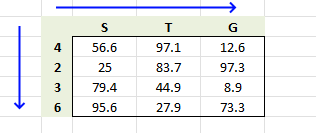The first condition is used in the horizontal lookup, the second condition is used in the vertical lookup.

#### Step 5 - Build LAMBDA function

The LAMBDA function build custom functions without VBA, macros or javascript.

Function syntax: LAMBDA([parameter1, parameter2, …,] calculation)

LAMBDA(a,INDEX(INDIRECT(a),MATCH(D23,INDEX(INDIRECT(a),1,0),0),MATCH(D24,INDEX(INDIRECT(a),0,1),0)))

The LAMBDA function is a requirement in order to use the MAP function in step 6.

#### Step 6 - Pass cell refs to LAMBDA function

The MAP function passes all values in an array to a LAMBDA function, the LAMBDA function calculates new values based on a formula you specify. It then returns an array with the same size as the original array.

Function syntax: MAP(array1, lambda_or_array<#>)

MAP(D19:D21,LAMBDA(a,INDEX(INDIRECT(a),MATCH(D23,INDEX(INDIRECT(a),1,0),0),MATCH(D24,INDEX(INDIRECT(a),0,1),0))))

The MAP function allows you to iterate or loop through each cell reference and return a corresponding value.

### Get the Excel fileCross-reference-multiple-tablesv2-Excel-365.xlsx

## 2. Two-way lookup using multiple tables - User Defined Function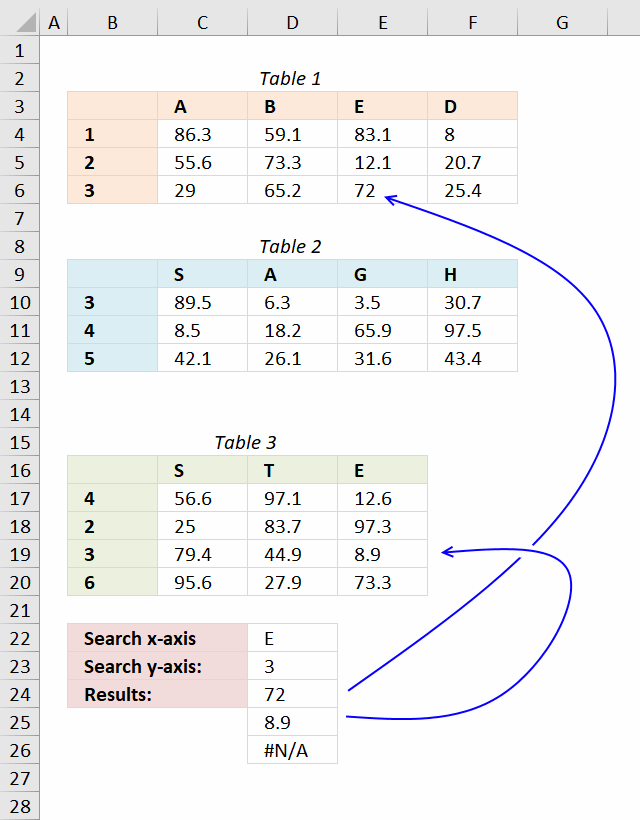A User defined function is a custom function anyone can use, simply copy the VBA code and paste to your workbooks code module and you are good to go.

The custom function demonstrated in this article accepts an arbitrary number of range arguments (table ranges). The custom function returns the first match in each table if there is a match.

Array formula in cell C24:C26:

=SearchMultipleTbl(D22,D23,B3:F6,B9:F12,B16:E20)

How to enter an array formula

1. Select cell range C24:C26
2. Copy above formula
3. Paste formula
4. Press and hold Ctrl + Shift
5. Press Enter once
6. Release all keys

### User Defined Function Syntax

SEARCHMULTIPLETBL(xaxis, yaxis, cellrange1, [cellrange2])

### Arguments

 Parameter Text xaxis Required. The x-axis condition you want to search for. yaxis Required. The y-axis condition you want to search for. cellrange1 Required. The range you want to add. [cellrange2] Optional. You may have up to 255 additional argument cell ranges.

VBA Code:

```'Name function and arguments
Function SEARCHMULTIPLETBL(xaxis As Variant, yaxis As Variant, ParamArray cellranges() As Variant)
'Declare variables and data types
Dim i As Integer, x As Variant, y As Variant
Dim temp() As Variant, xrange As Range, yrange As Range
'Redimension array variable temp so it may grow
ReDim temp(0)

'Iterate through all cell ranges
For i = LBound(cellranges) To UBound(cellranges)
'Enable error handling
On Error Resume Next
'Save first row to xrange object
Set xrange = cellranges(i).Rows(1)
'Save first column to yrange object
Set yrange = cellranges(i).Columns(1)
'Find position of matching value in row and column
x = Application.WorksheetFunction.Match(xaxis, xrange, 0)
y = Application.WorksheetFunction.Match(yaxis, yrange, 0)
'If found an error is not returned and Err.Number becomes 0 (zero)
If Err.Number = 0 Then
temp(UBound(temp)) = cellranges(i).Rows(y).Columns(x).Value
ReDim Preserve temp(UBound(temp) + 1)
Else
'Disable error handling
On Error GoTo 0
End If
'Continue with next cell range
Next i
'Remove last container in array
ReDim Preserve temp(UBound(temp) - 1)
'Return values in temp array rearranged (transposed)
SEARCHMULTIPLETBL= Application.Transpose(temp)

End Function
```

Where to copy vba code?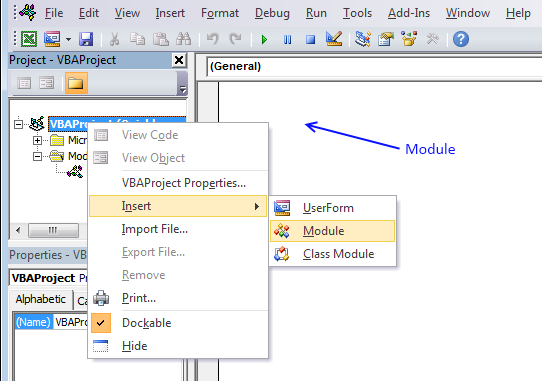1. Press Alt-F11 to open the Visual Basic Editor
2. Press with left mouse button on Module on the Insert menu
3. Copy and paste vba code.
4. Exit visual basic editor

### Get the Excel fileCross-reference-multiple-tablesv2.xlsm